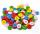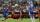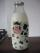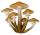Three workers

Three workers were rewarded CZK 9200 and the money divided by the work they have done. First worker to get twice than the second, the second three times more than the third. How much money each worker received?

Result

a =  5520
b =  2760
c =  920

Solution:

a+b+c = 9200
a = 2b
b = 3c

a+b+c = 9200
a-2b = 0
b-3c = 0

a = 5520
b = 2760
c = 920

Calculated by our linear equations calculator.

Leave us a comment of this math problem and its solution (i.e. if it is still somewhat unclear...):Be the first to comment!To solve this verbal math problem are needed these knowledge from mathematics:

Do you have a linear equation or system of equations and looking for its solution? Or do you have quadratic equation?

Next similar math problems:

1. Family parcelsIn father will he divided the land so that the older son had three bigger part than younger son. Later elder son gave 2.5 ha field to younger and they had both the same. Determine the area of family parcel.
2. Apples 2How many apples were originally on the tree, if the first day fell one third, the second day quarter of the rest and on tree remained 45 apples?
3. TreesAlong the road were planted 250 trees of two types. Cherry for 60 CZK apiece and apple 50 CZK apiece. The entire plantation cost 12,800 CZK. How many was cherries and apples?
4. ButtonsShirt has 6 buttons, blouse has 4 buttons. All buttons are 176. Shirts and blouses are together 36. How many are the shirts and blouses?
5. Fifth of the numberThe fifth of the number is by 24 less than that number. What is the number?
6. Spain vs USASpain lost to the US by 4 goals. In the match total fell 10 goals. How many goals gave the Spain and how the United States?
7. Simple equationSolve for x: 3(x + 2) = x - 18
8. Football leagueIn the 2nd football league plays each of the 16 participants with each opponent twice. For every victory gets 3 points, 1 point for a draw, a defeat none. After the competition was team with 54 points and 12 defeats at 9th place. How many times have won?
9. Two numbersWe have two numbers. Their sum is 140. One-fifth of the first number is equal to half the second number. Determine those unknown numbers.
10. Magic numberThe number 135 split to two addends so that one addend was 30 greater than 2/5 the addend.
11. The largerThe larger of two numbers is nine more than four times the smaller number. The sum of the two numbers is fifty-nine. Find the two numbers.
12. TipplerBottle with cork cost 8.8 Eur. The bottle is 0.8 euros more expensive than cork. How much is a bottle and the cork?
13. Football match 4In a football match with the Italy lost 3 goals with Germans. Totally fell 5 goals in the match. Determine the number of goals of Italy and Germany.
14. Unknown numberIdentify unknown number which 1/5 is 40 greater than one tenth of that number.
15. Belgium vs ItalyBelgium played a match with Italy and Belgium win by 2 goals. The match fell a total 6 goals. Determine the number of goals scored by Belgium and by Italy.
16. The dormitoryThe dormitory accommodates 150 pupils in 42 rooms, some of which are triple and some are quadruple. Determine how many rooms are triple and how many quadruples.
17. MushroomsFor five days, we have collected 410 mushrooms. Interestingly every day we have collected 10 mushrooms more than the preceding day. How many mushrooms we have collected during 4th day?